Courses

# NCERT Solutions(Part - 2) - Perimeter and Area Class 7 Notes | EduRev

## Mathematics (Maths) Class 7

Created by: Praveen Kumar

## Class 7 : NCERT Solutions(Part - 2) - Perimeter and Area Class 7 Notes | EduRev

The document NCERT Solutions(Part - 2) - Perimeter and Area Class 7 Notes | EduRev is a part of the Class 7 Course Mathematics (Maths) Class 7.
All you need of Class 7 at this link: Class 7

EXERCISE 11.3

Q.1. Find the circumference of the circles with the following radius: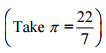(a) 14 cm

(b) 28 mm

(c) 21 cm

Solution.

(a) A circumference of the circle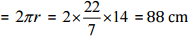(b) A circumference of the circle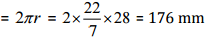(c) A circumference of the circle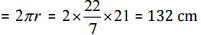Q.2. Find the area of the following circles, given that:(b) diameter = 49 m

Solution.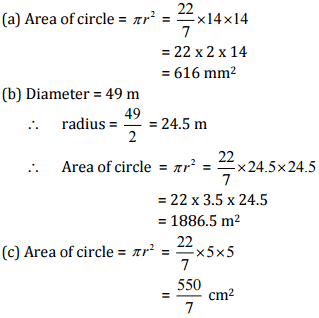Q.3. If the circumference of a circular sheet is 154 m, find its radius. Also, find the area of the sheet.Solution.
Circumference of the circular sheet = 154 m
⇒ 2πr = 154 m
⇒ r = 154/2π
⇒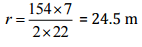Now, Area of circular sheet =πr2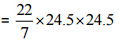= 22 x 3.5 x 24.5 = 1886.5 m2

Thus, the radius and area of circular sheet are 24.5 m and 1886.5 m2 respectively.

Q.4. A gardener wants to fence a circular garden of diameter 21 m. Find the length of the rope he needs to purchase if he makes 2 rounds of a fence. Also, find the costs of the rope, if it cost Rs 4 per meter.Solution.

Diameter of the circular garden = 21 m

∴ Radius of the circular garden = 21/2 m

Now, Circumference of a circular garden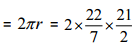= 22 x 3 = 66 m

The gardener makes 2 rounds of a fence so the total length of the rope of fencing
= 2 x 2πr
= 2 x 66 = 132 m

Since, the cost of 1 meter rope = Rs 4
Therefore, Cost of 132 meter rope = 4 x 132 = Rs 528

Q.5. From a circular sheet of radius 4 cm, a circle of radius 3 cm is removed. Find the area of the remaining sheet.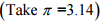Solution.

Radius of circular sheet (R) = 4 cm and radius of removed circle (r) = 3 cm
Area of the remaining sheet = Area of a circular sheet - Area of removed circle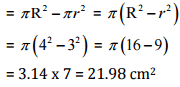Thus, the area of the remaining sheet is 21.98 cm2.

Q.6. Saima wants to put lace on the edge of a circular table cover of diameter 1.5 m. Find the length of the lace required and also find its cost if one meter of the lace costs Rs 15.Solution.

Diameter of the circular table cover = 1.5 m

∴ Radius of the circular table cover = 1.5/2 m

Circumference of circular table cover = 2πr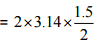= 4.71 m

Therefore, the length of required lace is 4.71 m.
Now the cost of 1 m lace = Rs 15
Then the cost of 4.71 m lace = 15 x 4.71 = Rs 70.65
Hence, the cost of 4.71 m lace is Rs 70.65.

Q.7. Find the perimeter of the adjoining figure, which is a semicircle including its diameter.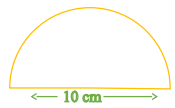Solution.

Diameter = 10 cm

Radius = 10/2 = 5 cm

According to question,
Perimeter of figure = Circumference of semicircle + diameter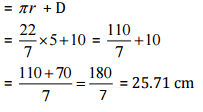Thus, the perimeter of the given figure is 25.71 cm.

Q.8. Find the cost of polishing a circular table-top of diameter 1.6 m, if the rate of polishing is Rs 15/m2. (Take π = 3.14)

Solution.
Diameter of the circular table top = 1.6 m

Radius of the circular table top = 1.6/2 = 0.8 m

Area of circular table top = πr2 = 3.14 x 0.8 x 0.8 = 2.0096 m2
Now, the cost of polishing 1 m2 = Rs 15
Then cost of polishing 2.0096 m2 = 15 x 2.0096 = Rs 30.14 (approx.)
Thus, The cost of polishing a circular table top is Rs 30.14 (approx.)

Q.9. Shazli took a wire of length 44 cm and bent it into the shape of a circle. Find the radius of that circle. Also, find its area. If the same wire is bent into the shape of a square, what will be the length of each of its sides? Which figure encloses more area, the circle or the square?Solution.

Total length of the wire = 44 cm
∴ The circumference of the circle = 2πr = 44 cm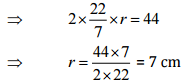Now Area of the circle = πr2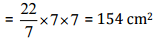Now the wire is converted into the square.
The perimeter of square = 44 cm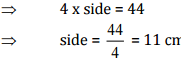Now, area of square = side x side = 11 x 11 = 121 cm2

Therefore, on comparing, the area of the circle is greater than that of a square, so the circle encloses more area.

Q.10. From a circular card sheet of radius 14 cm, two circles of radius 3.5 cm and a rectangle of length 3 cm and breadth 1 cm are removed (as shown in the adjoining figure). Find the area of the remaining sheet.Solution.

Radius of circular sheet (R) = 14 cm and Radius of smaller circle (r) = 3.5 cm
Length of rectangle (l) = 3 cm and breadth of rectangle (b) = 1 cm
According to question,
Area of remaining sheet = Area of circular sheet- (Area of two smaller circle + Area of rectangle)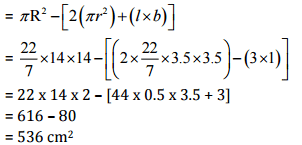Therefore, the area of the remaining sheet is 536 cm2.

Q.11. A circle of radius 2 cm is cut out from a square piece of an aluminum sheet of side 6 cm. What is the area of the leftover aluminum sheet?Solution.

Radius of circle = 2 cm and side of aluminium square sheet = 6 cm
According to question,
Area of aluminum sheet left = Total area of aluminum sheet - Area of circle
= side x side - πr2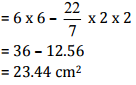Therefore, the area of the aluminum sheet left is 23.44 cm2.

Q.12. The circumference of a circle is 31.4 cm. Find the radius and the area of the circle.Solution.

The circumference of the circle = 31.4 cm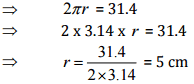Then area of the circle = πr2 = 3.14 x 5 x 5
= 78.5 cm2
Therefore, the radius and the area of the circle are 5 cm and 78.5 cm2 respectively.

Q.13. A circular flower bed is surrounded by a path 4 m wide. The diameter of the flower bed is 66 m. What is the area of this path?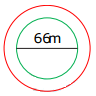Solution.

Diameter of the circular flower bed = 66 m
∴ Radius of circular flower bed (r) = 66/2 = 33 m
∴ Radius of circular flower bed with 4 m wide path (R) = 33 + 4 = 37 m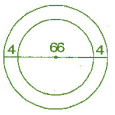According to question,
Area of path = Area of bigger circle - Area of smaller circle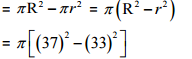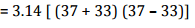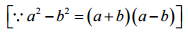= 3.14 x 70 x 4
= 879.20 m2
Therefore, the area of the path is 879.20 m2.

Q.14. A circular flower garden has an area of 314 m2. A sprinkler at the centre of the garden can cover an area that has a radius of 12 m. Will the sprinkler water the entire garden?Solution.

Circular area covered by the sprinkler = πr2
= 3.14 x 12 x 12
= 3.14 x 144
= 452.16 m2
Area of the circular flower garden = 314 m2

As Area of the circular flower garden is smaller than area with a sprinkler. Therefore, the sprinkler will water the entire garden.

Q.15. Find the circumference of the inner and the outer circles, shown in the adjoining figure.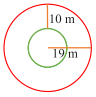Solution.

Radius of outer circle (r) = 19 m
Circumference of outer circle = 2πr = 2 x 3.14 x 19 = 119.32 m
Now radius of inner circle (r')  = 19 - 10 = 9 m
∴ Circumference of inner circle = 2πr' = 2 x 3.14 x 9 = 56.52 m

Therefore, the circumferences of inner and outer circles are 56.52 m and 119.32 m respectively.

Q.16. How many times a wheel of radius 28 cm must rotate to go 352 m?Solution.

Let wheel must be rotated n times of its circumference.
Radius of wheel = 28 cm and Total distance = 352 m = 35200 cm
∴ Distance covered by wheel = n x circumference of wheel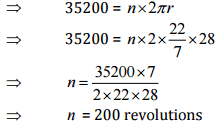Thus, the wheel must rotate 200 times to go 352 m.

Q.17. The minute hand of a circular clock is 15 cm long. How far does the tip of the minute hand move in 1 hour?Solution.

In 1 hour, minute hand completes one round means making a circle.
Radius of the circle (r) = 15 cm
A circumference of circular clock = 2πr
= 2 x 3.14 x 15
= 94.2 cm

Therefore, the tip of the minute hand moves 94.2 cm in 1 hour.

Offer running on EduRev: Apply code STAYHOME200 to get INR 200 off on our premium plan EduRev Infinity!

,

,

,

,

,

,

,

,

,

,

,

,

,

,

,

,

,

,

,

,

,

;